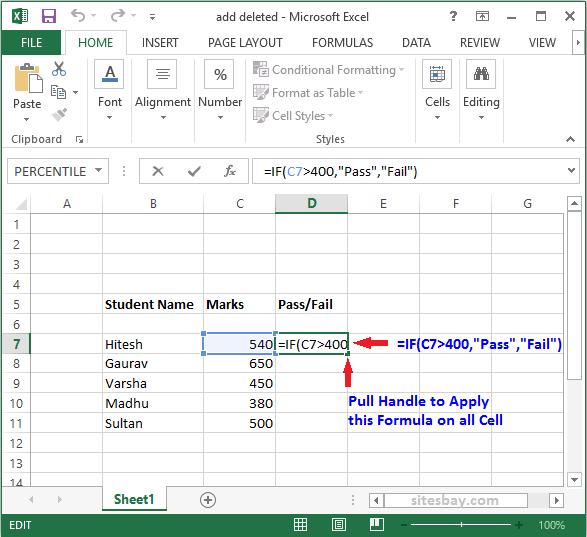# Excel If Function

## If Function in Excel

The IF function or IF statement in Excel is composed of three parts separated by commas.

• Condition
• What to display if the condition is true
• What to display if the condition is false

See in below image how to display pass and fail student if marks is greater than 400 it display pass otherwise fail.### How to Create If Function in Excel

To create If function in excel follow below steps;

• Type the code in the cell: =if(
• Type the condition with comma: C7>70,
• Type what you want to show if condition is true. Here we display Pass if condition is true
• Type a comma: ,
• Type what you want to show if condition is false. Here we display Fail if condition is false
• Then close the bracket and press the Enter key.
• Final function look like this =IF(C7>400,"Pass","Fail")

### If Condition Apply on Cell

Some time we need to print specific cell value based on condition,use below syntax.

## Syntax

```=IF(C7>10,A1,A1+10)
```

Using above syntax we print value of cell A1 if condition is true otherwise print cell A1+10